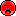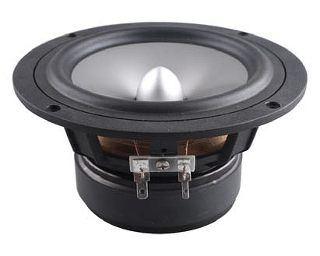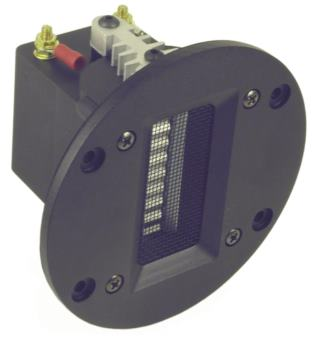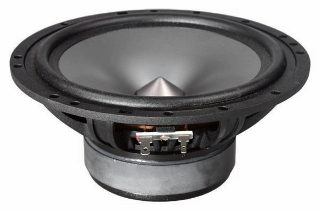# Fountek

Fountek is an innovative far east manufacturer of ribbon tweeters. The product quality (even mechanical details like the neat rear damping coat of the front plate), the powerful Neodym magnets, the high efficiency and the flat frequency response convinced us. Therefore, selected pairs of ribbon tweeters with metal front plate (Fountek also supplies common types with plastic front plate) are available under the name Audaphon/Fountek.

The following ribbon tweeters are so-called real ribbons, with built-in transformer, since ribbons of around 0.02 Ohm have a too low resistance to be wired up directly.

There are two different materials for ribbons: 0.009 mm aluminium foil, like the JP ribbons These ribbons show an incredible airiness; they need, however, to be protected carefully against low frequencies, by means of 18 dB crossover and should never be connected directly (without capacitor) to a measuring device.

The next type of ribbon is the Neo range with a stronger ribbon that is roughly 50% heavier and has with a 0.020 mm gauge double the thickness. These could be used in conjunction with 12 dB crossovers.

Particularly impressive is the Neo 5i ribbon, equipped with 4 high power Neodym magnets, totalling a weight of 2.7 kg.

## Ribbon Tweeters## Fountek Neo CD 2.0 silver

order no. fo-cd20- not available

ribbon tweeter.

• frequency range = 1200-40000 Hz
• resonance frequency fs = 350 Hz
• impedance R = 7 Ohm
• sound pressure level SPL = 92 dB (2,83V; 1m)
• DC resistance Re = 7 Ohm
• effective piston radiating area Sd = 9,6 cm2
• effective mechanical mass incl. air load mms = 0,034 g
• overall diameter d = 164 x 70,4 mm
• mounting depth (not countersunk) t = 70 mm
PDF data sheet## Fountek Neo CD 2.0 black

order no. fo-cd20b

EUR 199,00excl. VAT: € 167.23 / \$ 177.26

ribbon tweeter.

• frequency range = 1200-40000 Hz
• resonance frequency fs = 350 Hz
• impedance R = 7 Ohm
• sound pressure level SPL = 92 dB (2,83V; 1m)
• DC resistance Re = 7 Ohm
• effective piston radiating area Sd = 9,6 cm2
• effective mechanical mass incl. air load mms = 0,034 g
• overall diameter d = 164 x 70,4 mm
• mounting depth (not countersunk) t = 70 mm
PDF data sheet## Fountek JP 2.0

order no. fo-jp20- not available

ribbon tweeter.

• frequency range = 1700-40000 Hz
• resonance frequency fs = 370 Hz
• impedance R = 7 Ohm
• sound pressure level SPL = 93 dB (2,83V; 1m)
• DC resistance Re = 7 Ohm
• effective piston radiating area Sd = 9,6 cm2
• effective mechanical mass incl. air load mms = 0,025 g
• overall diameter d = 164 x 70,4 mm
• mounting depth (not countersunk) t = 70 mm## Fountek Neo Pro 5i

order no. fo-pro5i

EUR 399,00excl. VAT: € 335.29 / \$ 355.41

ribbon tweeter.

• frequency range = 650-40000 Hz
• resonance frequency fs = 200 Hz
• impedance R = 7 Ohm
• sound pressure level SPL = 96 dB (2,83V; 1m)
• DC resistance Re = 5,15 Ohm
• effective piston radiating area Sd = 21,75 cm2
• effective mechanical mass incl. air load mms = 0,088 g
• overall diameter d = 190 x 115 mm
• mounting depth (not countersunk) t = 99 mm
PDF data sheet

## Full Range## Fountek FE87

order no. fo-fe87

EUR 14,10excl. VAT: € 11.85 / \$ 12.56

7 cm full range driver with aluminium cone. The driver is magnetically shielded.

• power handling (continuous/programme) = 13 W
• frequency range = 100-25000 Hz
• resonance frequency fs = 101 Hz
• impedance R = 8 Ohm
• sound pressure level SPL = 84 dB (2,83V; 1m)
• DC resistance Re = 6,776 Ohm
• force factor BL = 3,1 N/A
• effective piston radiating area Sd = 27,34 cm2
• effective mechanical mass incl. air load mms = 2,073 g
• equivalent volume of compliance Vas = 1,254 l
• total Q factor Qts = 0,73 (Qms=3,381, Qes=0,939)
• voice coil diameter = 25 mm
• maximum peak linear excursion vibration xlin = +/- 1,0 mm
• mounting diameter d = 76,5 mm
• overall diameter d = 79 mm
• mounting depth (not countersunk) t = 52,7 mm
• mass m = 0,56 kg
PDF data sheet

## Fountek FR88

order no. fo-fr88- not available

8 cm full range driver with aluminium cone.

• power handling (continuous/programme) = 15 W
• frequency range = 105-25000 Hz
• resonance frequency fs = 105 Hz
• impedance R = 8 Ohm
• sound pressure level SPL = 85 dB (2,83V; 1m)
• DC resistance Re = 6,3 Ohm
• force factor BL = 3,152 N/A
• effective piston radiating area Sd = 27,34 cm2
• effective mechanical mass incl. air load mms = 2 g
• equivalent volume of compliance Vas = 1,2 l
• total Q factor Qts = 0,6 (Qms=2,568, Qes=0,777)
• voice coil diameter = 25 mm
• maximum peak linear excursion vibration xlin = +/- 1 mm
• mounting diameter d = 73,9 mm
• overall diameter d = 88,4 mm
• mounting depth (not countersunk) t = 48,1 mm
• mass m = 0,58 kg
PDF data sheetopen a bigger photo

recommended cabinet 1:
closed cabinet with 0,48 L volume
from 167/105 Hz (-3dB/-8dB)

recommended cabinet 2:
1,9 L volume bass reflex cabinet
with 2cm-Rohr reflex tube, 6 cm long.
from 79/65 Hz (-3dB/-8dB).

## Fountek FR89EX

order no. fo-fr89EX- not available

8 cm full range driver with aluminium cone. The magnet system is based on Neodymium technology.

• power handling (continuous/programme) = 25 W
• frequency range = 70-2000 Hz
• resonance frequency fs = 97 Hz
• impedance R = 8 Ohm
• sound pressure level SPL = 83,6 dB (2,83V; 1m)
• DC resistance Re = 5,7 Ohm
• force factor BL = 3,781 N/A
• effective piston radiating area Sd = 27,34 cm2
• effective mechanical mass incl. air load mms = 2,611 g
• equivalent volume of compliance Vas = 1,111 l
• total Q factor Qts = 0,47 (Qms=1,642, Qes=0,652)
• voice coil diameter = 25 mm
• maximum peak linear excursion vibration xlin = +/- 5 mm
• mounting diameter d = 73 mm
• overall diameter d = 88,4 mm
• mounting depth (not countersunk) t = 46,9 mm
• mass m = 0,525 kg
PDF data sheetopen a bigger photo

recommended cabinet 1:
closed cabinet with 2,9 L volume
from 98/62 Hz (-3dB/-8dB)

recommended cabinet 2:
10,9 L volume bass reflex cabinet
with HP50 reflex tube, 16 cm long.
from 48/39 Hz (-3dB/-8dB).

## Fountek FW146

order no. fo-fw146

EUR 53,70excl. VAT: € 45.13 / \$ 47.83

13 cm bass-midrange driver with aluminium cone.

• power handling (continuous/programme) = 35 W
• frequency range = 45-4000 Hz
• resonance frequency fs = 50 Hz
• impedance R = 8 Ohm
• sound pressure level SPL = 86,1 dB (2,83V; 1m)
• DC resistance Re = 6 Ohm
• force factor BL = 6,065 N/A
• effective piston radiating area Sd = 77,61 cm2
• effective mechanical mass incl. air load mms = 8,936 g
• equivalent volume of compliance Vas = 9,8 l
• total Q factor Qts = 0,4 (Qms=3,508, Qes=0,456)
• voice coil diameter = 25 mm
• maximum peak linear excursion vibration xlin = +/- 3,6 mm
• mounting diameter d = 115 mm
• overall diameter d = 146 mm
• mounting depth (not countersunk) t = 68,7 mm
• mass m = 1,265 kg
PDF data sheet

## Fountek NeoCD1.0

order no. fo-cd10- not available

ribbon tweeter. The magnet system is based on Neodymium technology.

• power handling (continuous/programme) = 12/25 W
• frequency range = 2000-40000 Hz
• impedance R = 5 Ohm
• sound pressure level SPL = 90 dB (2,83V; 1m)
• DC resistance Re = 0,02 Ohm
• effective piston radiating area Sd = 3,04 cm2
• effective mechanical mass incl. air load mms = 0,10 g
• mounting depth (not countersunk) t = 70 mm
• mass m = 0,41 kg
PDF data sheet

## Fountek NeoCD3.5H

order no. fo-cd35- not available

ribbon tweeter. The magnet system is based on Neodymium technology.

• power handling (continuous/programme) = 12/25 W
• frequency range = 1100-30000 Hz
• impedance R = 7 Ohm
• sound pressure level SPL = 95,5 dB (2,83V; 1m)
• DC resistance Re = 0,02 Ohm
• effective piston radiating area Sd = 3,60 cm2
• effective mechanical mass incl. air load mms = 0,30 g
• mounting depth (not countersunk) t = 101 mm
• mass m = 0,52 kg
PDF data sheet## Fountek Neo CD 3.0

order no. fo-cd30- not available

ribbon tweeter.

• frequency range = 1400-40000 Hz
• resonance frequency fs = 400 Hz
• impedance R = 7 Ohm
• sound pressure level SPL = 90 dB (2,83V; 1m)
• DC resistance Re = 7 Ohm
• effective piston radiating area Sd = 4,8 cm2
• effective mechanical mass incl. air load mms = 0,018 g
• mounting diameter d = 90 mm
• overall diameter d = 110 mm
• mounting depth (not countersunk) t = 68 mm
PDF data sheet

## no longer sold## Fountek JP 3.0

order no. fo-jp30- not available

ribbon tweeter.

• frequency range = 1700-40000 Hz
• resonance frequency fs = 220 Hz
• impedance R = 7 Ohm
• sound pressure level SPL = 91 dB (2,83V; 1m)
• DC resistance Re = 7 Ohm
• effective piston radiating area Sd = 4,8 cm2
• effective mechanical mass incl. air load mms = 0,011 g
• mounting diameter d = 90 mm
• overall diameter d = 110 mm
• mounting depth (not countersunk) t = 68 mmopen a bigger photo

recommended cabinet 1:
closed cabinet with 3,2 L volume
from 99/63 Hz (-3dB/-8dB)

recommended cabinet 2:
13,3 L volume bass reflex cabinet
with HP50 reflex tube, 12 cm long.
from 46/39 Hz (-3dB/-8dB).

## Fountek FR135EX

order no. fo-fr135EX- not available

13 cm full range driver with aluminium cone. The magnet system is based on Neodymium technology.

• power handling (continuous/programme) = 40 W
• frequency range = 50-26000 Hz
• resonance frequency fs = 61,5 Hz
• impedance R = 8 Ohm
• sound pressure level SPL = 85,1 dB (2,83V; 1m)
• DC resistance Re = 6 Ohm
• force factor BL = 5,027 N/A
• effective piston radiating area Sd = 70,5 cm2
• effective mechanical mass incl. air load mms = 7,546 g
• equivalent volume of compliance Vas = 6,263 l
• total Q factor Qts = 0,49 (Qms=1,709, Qes=0,692)
• voice coil diameter = 32 mm
• maximum peak linear excursion vibration xlin = +/- 5 mm
• mounting diameter d = 115 mm
• overall diameter d = 145,5 mm
• mounting depth (not countersunk) t = 68,8 mm
• mass m = 0,98 kg
PDF data sheetopen a bigger photo

recommended cabinet 1:
closed cabinet with 0,47 L volume
from 198/125 Hz (-3dB/-8dB)

recommended cabinet 2:
1,9 L volume bass reflex cabinet
with HP35 reflex tube, 11 cm long.
from 91/77 Hz (-3dB/-8dB).

## Fountek FE85

order no. fo-fe85- not available

7 cm full range driver with aluminium cone. The magnet system is based on Neodymium technology.

• power handling (continuous/programme) = 12 W
• frequency range = 100-25000 Hz
• resonance frequency fs = 125 Hz
• impedance R = 8 Ohm
• sound pressure level SPL = 85,7 dB (2,83V; 1m)
• DC resistance Re = 6 Ohm
• force factor BL = 3,71 N/A
• effective piston radiating area Sd = 27 cm2
• effective mechanical mass incl. air load mms = 2,07 g
• equivalent volume of compliance Vas = 0,85 l
• total Q factor Qts = 0,51 (Qms=2,13, Qes=0,67)
• voice coil diameter = 20 mm
• maximum peak linear excursion vibration xlin = +/- 1,5 mm
• mounting diameter d = 70 mm
• overall diameter d = 83,5 mm
• mounting depth (not countersunk) t = 43,7 mm
• mass m = 0,47 kg
PDF data sheetopen a bigger photo

recommended cabinet 1:
closed cabinet with 0,58 L volume
from 160/101 Hz (-3dB/-8dB)

recommended cabinet 2:
2,4 L volume bass reflex cabinet
with 2cm-Rohr reflex tube, 5 cm long.
from 75/63 Hz (-3dB/-8dB).

## Fountek FR88EX

order no. fo-fr88EX- not available

8 cm full range driver with aluminium cone. The magnet system is based on Neodymium technology.

• power handling (continuous/programme) = 15 W
• frequency range = 95-20000 Hz
• resonance frequency fs = 95 Hz
• impedance R = 8 Ohm
• sound pressure level SPL = 84,3 dB (2,83V; 1m)
• DC resistance Re = 6 Ohm
• force factor BL = 3,313 N/A
• effective piston radiating area Sd = 27,34 cm2
• effective mechanical mass incl. air load mms = 2,356 g
• equivalent volume of compliance Vas = 1,269 l
• total Q factor Qts = 0,47 (Qms=1,96, Qes=0,622)
• voice coil diameter = 25 mm
• maximum peak linear excursion vibration xlin = +/- 3 mm
• mounting diameter d = 73 mm
• overall diameter d = 88,4 mm
• mounting depth (not countersunk) t = 38 mm
• mass m = 0,35 kg
PDF data sheetopen a bigger photo

recommended cabinet 1:
closed cabinet with 10,9 L volume
from 81/51 Hz (-3dB/-8dB)

recommended cabinet 2:
44 L volume bass reflex cabinet
with HP70 reflex tube, 9 cm long.
from 38/32 Hz (-3dB/-8dB).

## Fountek FW166

order no. fo-fw166- not available

15 cm bass-midrange driver with aluminium cone.

• power handling (continuous/programme) = 30 W
• frequency range = 46-2500 Hz
• resonance frequency fs = 46 Hz
• impedance R = 4 Ohm
• sound pressure level SPL = 89 dB (2,83V; 1m)
• DC resistance Re = 3,4 Ohm
• force factor BL = 4,827 N/A
• effective piston radiating area Sd = 136,85 cm2
• effective mechanical mass incl. air load mms = 11,947 g
• equivalent volume of compliance Vas = 27,13 l
• total Q factor Qts = 0,44 (Qms=3,623, Qes=0,499)
• voice coil diameter = 25 mm
• maximum peak linear excursion vibration xlin = +/- 3,1 mm
• mounting diameter d = 145 mm
• overall diameter d = 166 mm
• mounting depth (not countersunk) t = 70,6 mm
• mass m = 1,215 kg
PDF data sheetopen a bigger photo

recommended cabinet 1:
closed cabinet with 4,5 L volume
from 90/57 Hz (-3dB/-8dB)

recommended cabinet 2:
17 L volume bass reflex cabinet
with HP70 reflex tube, 24 cm long.
from 45/36 Hz (-3dB/-8dB).

## Fountek FW168

order no. fo-fw168- not available

16 cm bass-midrange driver with aluminium cone.

• power handling (continuous/programme) = 50 W
• frequency range = 40-4000 Hz
• resonance frequency fs = 45 Hz
• impedance R = 8 Ohm
• sound pressure level SPL = 87,3 dB (2,83V; 1m)
• DC resistance Re = 7,2 Ohm
• force factor BL = 8,2 N/A
• effective piston radiating area Sd = 119 cm2
• effective mechanical mass incl. air load mms = 14,7 g
• equivalent volume of compliance Vas = 16,5 l
• total Q factor Qts = 0,4 (Qms=3,246, Qes=0,452)
• voice coil diameter = 36 mm
• maximum peak linear excursion vibration xlin = +/- 4,6 mm
• mounting diameter d = 146 mm
• overall diameter d = 168 mm
• mounting depth (not countersunk) t = 75 mm
• mass m = 1,76 kg
PDF data sheetopen a bigger photo

recommended cabinet 1:
closed cabinet with 39 L volume
from 54/34 Hz (-3dB/-8dB)

recommended cabinet 2:
158 L volume bass reflex cabinet
with HP100 reflex tube, 11 cm long.
from 25/21 Hz (-3dB/-8dB).

## Fountek FW200

order no. fo-fw200- not available

20 cm bass-midrange driver with aluminium cone.

• power handling (continuous/programme) = 75 W
• frequency range = 35-3000 Hz
• resonance frequency fs = 35,7 Hz
• impedance R = 8 Ohm
• sound pressure level SPL = 88 dB (2,83V; 1m)
• DC resistance Re = 6,8 Ohm
• force factor BL = 6,83 N/A
• effective piston radiating area Sd = 213,8 cm2
• effective mechanical mass incl. air load mms = 20,927 g
• equivalent volume of compliance Vas = 61,6 l
• total Q factor Qts = 0,52 (Qms=2,258, Qes=0,683)
• voice coil diameter = 36 mm
• maximum peak linear excursion vibration xlin = +/- 4,35 mm
• mounting diameter d = 185 mm
• overall diameter d = 217 mm
• mounting depth (not countersunk) t = 88,8 mm
• mass m = 1,9 kg
PDF data sheet

## Woofer## Fountek FW300 (single coil)

order no. fo-fw300- not available

29 cm bass driver with aluminium cone.

• power handling (continuous/programme) = 600 W
• frequency range = 31-1000 Hz
• resonance frequency fs = 37,247 Hz
• impedance R = 8 Ohm
• sound pressure level SPL = 86,2 dB (2,83V; 1m)
• DC resistance Re = 3,6 Ohm
• force factor BL = 11,392 N/A
• effective piston radiating area Sd = 530,93 cm2
• effective mechanical mass incl. air load mms = 145,782 g
• equivalent volume of compliance Vas = 50,132 l
• total Q factor Qts = 0,78 (Qms=4,557, Qes=0,946)
• voice coil diameter = 50 mm
• maximum peak linear excursion vibration xlin = +/- 14,25 mm
• mounting diameter d = 270,2 mm
• overall diameter d = 314 mm
• mounting depth (not countersunk) t = 166,4 mm
• mass m = 6,6 kg
PDF data sheetopen a bigger photo

recommended cabinet 1:
closed cabinet with 23 L volume
from 64/41 Hz (-3dB/-8dB)

recommended cabinet 2:
93 L volume bass reflex cabinet
with 2x HP100 reflex tube, 33 cm long.
from 30/25 Hz (-3dB/-8dB).

## Fountek FW300

order no. fo-fw300- not available

29 cm bass driver with aluminium cone.

• power handling (continuous/programme) = 600 W
• frequency range = 31-1000 Hz
• resonance frequency fs = 38,21 Hz
• impedance R = 8 Ohm
• sound pressure level SPL = 89,1 dB (2,83V; 1m)
• DC resistance Re = 7,2 Ohm
• force factor BL = 21,274 N/A
• effective piston radiating area Sd = 530,93 cm2
• effective mechanical mass incl. air load mms = 138,83 g
• equivalent volume of compliance Vas = 50,022 l
• total Q factor Qts = 0,47 (Qms=4,246, Qes=0,53)
• voice coil diameter = 50 mm
• maximum peak linear excursion vibration xlin = +/- 14,25 mm
• mounting diameter d = 270,2 mm
• overall diameter d = 314 mm
• mounting depth (not countersunk) t = 166,4 mm
• mass m = 6,6 kg
PDF data sheet

The cabinet volume calculations are executed considering an external resistance of 0.3 Ohm. We are using, if no other data is known or available, the information supplied by the manufacturer.

Please note: the frequency response doesn't say much about the cut-off frequency inside the cabinet! Therefore, we mostly give you 2 examples with dimensioning considerations and an indication, how low the loudspeaker will reproduce.

Home